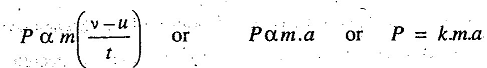# Mass, Weight, Momentum and Inertia

Define Mass, Weight, Momentum and Inertia Force in Engineering Mechanics.

The mass is a matter contained in a body. It is expressed in kilogram (kg).

The weight of a body is a force by which it is attracted towards the center of the earth. It is expressed in newtons (N). The relation between the mass and weight of a body is given by

W = m.g

The momentum is defined as the total Motion possessed by a body. Mathematically,

Momentum = Mass x Velocity

According to Newton's second law of motion, the applied force or impressed force (P) is directly proportional to rate of Change of momentum, Thuswhere k is a Constant of proportionality.

For the sake of convenience, the unit of force adopted is such that it produces unit acceleration (i.e. 1m/s2) to a body of unit mass (i.e. 1 kg).

P = m.a = Mass x Acceleration

In S.I system of units, the unit of force is newton (briefly written as N). A newton may be defined as the force, while acting upon a body of mass 1 kg, produces an acceleration of lm/s2 in the direction of which it acts. Thus

1N = 1kg x 1m/s2 = 1kg-m/s2

Note : A force equal in magnitude but opposite in direction and collinear with the applied force or impressed force producing the acceleration, is known as inertia force. Mathematically, inertia force,
FI= – m.a